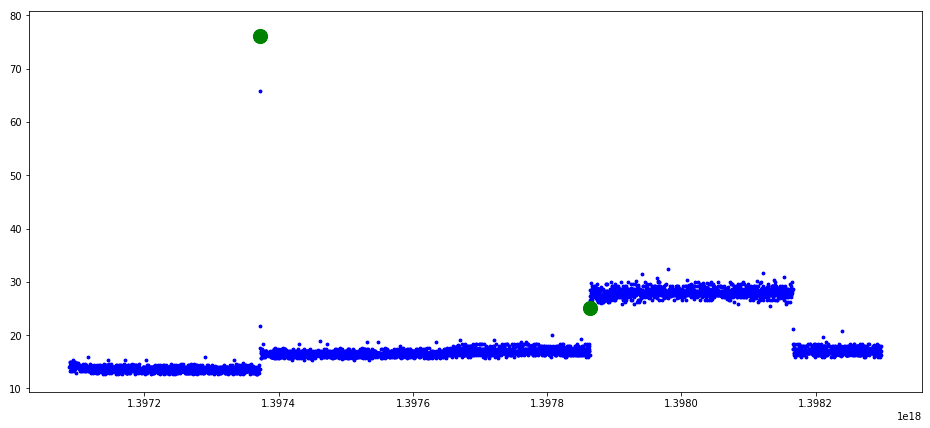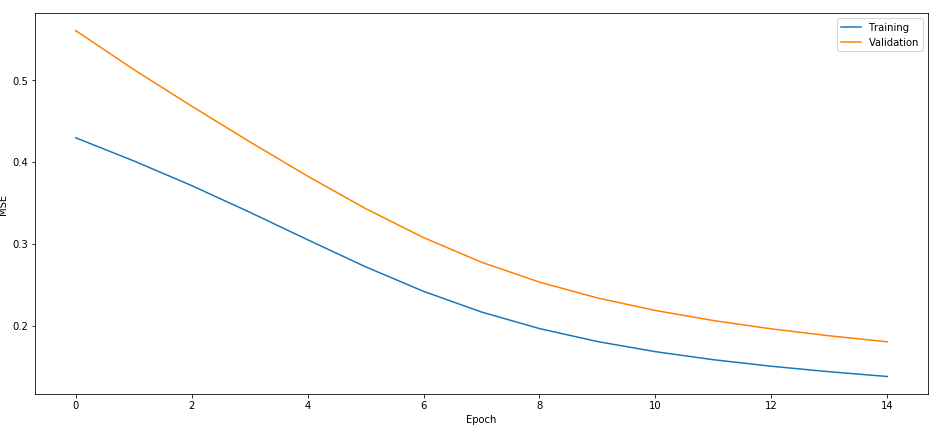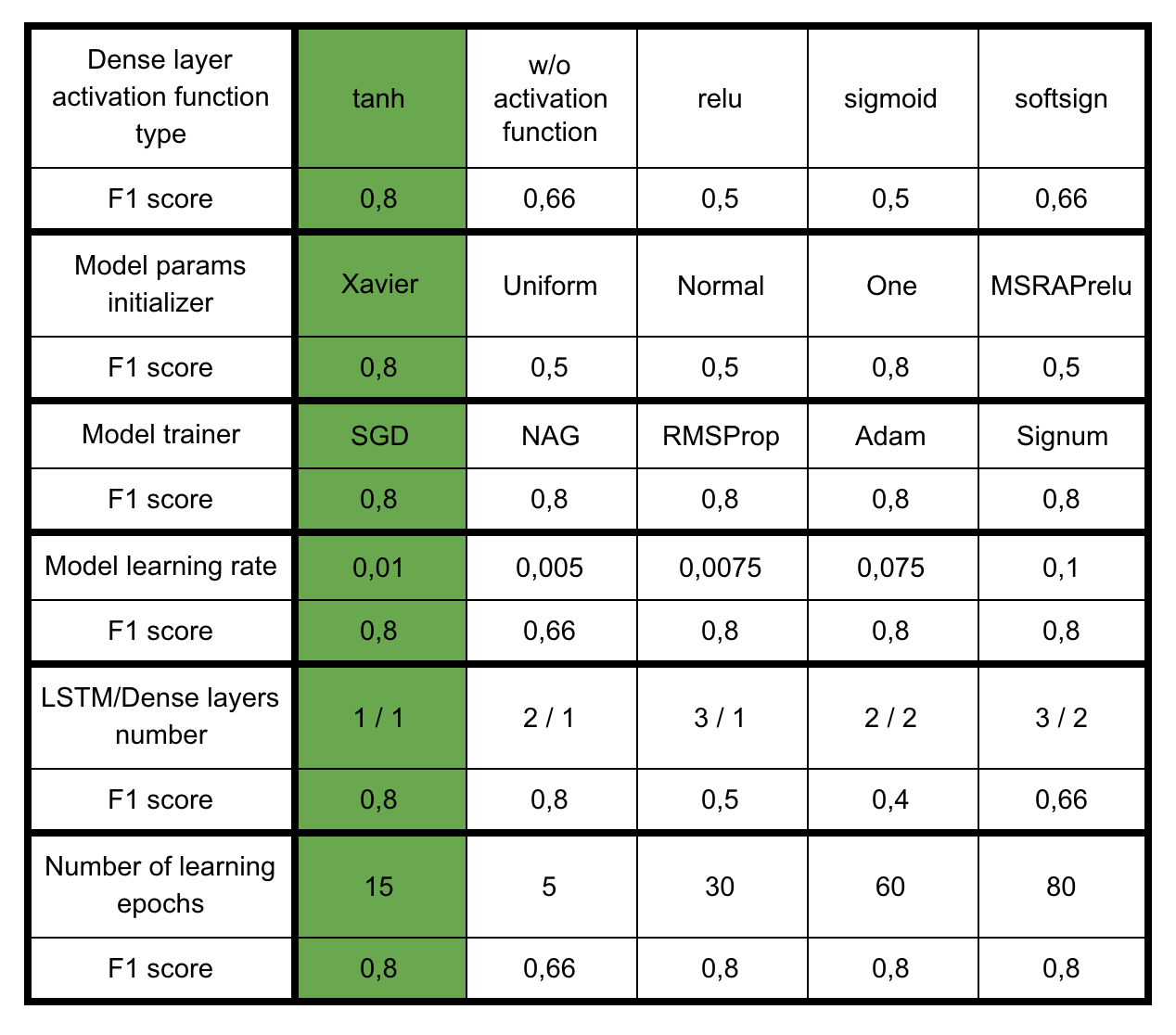# Time Series Anomaly Detection with LSTM and MXNet

17. December 2018 0

As software engineers, we try our best to make sure that the solution we build is reliable and robust. Monitoring the production environment with reasonable alerts and timely actions to mitigate and resolve any issues are pieces of the puzzle required to make our customers happy. Monitoring can produce a lot of data – CPU, memory, and disk IO are the most commonly collected for hardware infrastructure, however, in most cases, you never know what the anomaly is, so the data is not labeled.

We decided to take a common problem – anomaly detection within a time series data of CPU utilization and explore how to identify it using unsupervised learning. A dataset we use is the Numenta Anomaly Benchmark (NAB). It is labeled, and we will use labels for calculating scores and the validation set. There are plenty of well-known algorithms that can be applied for anomaly detection – K-nearest neighbor, one-class SVM, and Kalman filters to name a few. However, most of them do not shine in the time series domain. According to many studies  , long short-term memory (LSTM) neural network should work well for these types of problems.

TensorFlow is currently the trend leader in deep learning, however, at Lohika we have pretty good experience with another solid deep-learning framework, Apache MXNet. We like it because it is light, scalable, portable and well-documented, and it is also Amazon’s preferred choice for their deep-learning framework at AWS.

The neural network that we are going to implement is called autoencoder. The autoencoder is a type of neural network that calculates the approximation of the input function by transforming the input data to the intermediate state and then matching it against the number of input features. When training autoencoders the idea is to minimize some metric dependent on input and output values. We use MSE for the case. To compare the performance of different network designs and hyperparameters we use F1 score. F1 score conveys the balance between the precision and the recall and is commonly used for binary classification.

The goal for this task is to detect all known anomalies on the test set and get the maximum F1 score.

For the implementation, we use Python and a few libraries that are very handy – pandas for dataset manipulation, scikit-learn for data pre-processing and metrics calculations, and matplotlib for visualization.

So let’s get to it…

## Dataset Overview

The NAB dataset contains a lot of labeled real and artificial data that can be used for anomaly detection algorithm evaluation. We used actual CPU utilization data of some AWS RDS instances for our study. The dataset contains 2 files of records with the values taken every 5 minutes for a period of 14 days, 4032 entities for each file. We used one file for training and another for test purposes.

Deep learning requires large amounts of data for real-world applications. But smaller datasets are acceptable for basic study, especially since model training doesn’t take much time.

Let’s describe all paths to datasets and labels:

Anomaly labels are stored separately from the data values. Let’s load the train and test datasets and label the values with pandas:As we can see, it contains a timestamp, a CPU utilization value, and labels noting if this value is an anomaly.

The next step is a visualization of the dataset with pyplot, which requires converting timestamps to time epochs:

When plotting the data, we mark anomalies with green dots:

The visualization of the training and test datasets look like this:## Preparing the Dataset

There is one thing that played an important role in dataset preparation – masking of labeled anomalies.

We started with training our LSTM neural network on the initial dataset. This resulted in our model being able to predict, at best, one anomaly out of the 2 labeled in the test dataset. Then, after taking into account that we have a small dataset with limited anomalies, we decided to test if training on a dataset that contains no anomalies would improve results?

We took the approach of masking the anomalies in the original dataset – just put previous non-anomalous value instead of anomalies. After training the model on the dataset with masked anomalies, we were able to get both anomalies predicted. However, this was at the expense of an additional false positive prediction.

Nevertheless, the F1 score is higher for the second case, so the final implementation should take into account the preferred solution. In practice it can have a significant impact – depending on your case, both missing an anomaly or having a false positive prediction can be quite expensive.

Let’s prepare the data for the machine learning processing. The training set contains anomalies, as described above, replaced with non-anomalous values. The simplest way is to use pandas ‘fillna’ method with ‘ffill’ param to replace anomalies values with neighbors:

The next step is scaling the dataset values. This is highly important. We use scikit-learn StandardScaler to scale the input data and pandas to select features from the dataset.

The only feature we use is the CPU utilization value. We tried extracting some additional time-based features to increase the output performance – for example, a weekday or a day/night feature -however, we didn’t find any useful patterns this way.

Let’s prepare our training and validation datasets:

## Choosing a Model

Let’s define the neural network model for our autoencoder:

There is a lot happening in this small piece of code. Let’s review it line-by-line:

• `gluon.nn.Sequential()` stacks blocks sequentially
• `model.add` – adds a block to the top of the stack
• `gluon.rnn.LSTM(n)` – LSTM layer with n-output dimensionality. In our situation, we used an LSTM layer without dropout at the layer output. Commonly, dropout layers are used for preventing the overfitting of the model. It’s just zeroed the layer inputs with the given probability
• `gluon.nn.Dense(n, activation='tanh')` – densely-connected NN layer with n-output dimensionality and hyperbolic tangent activation function

We did a few experiments with the neural network architecture and hyperparameters, and the LSTM layer followed by one Dense layer with ‘tanh’ activation function worked best in our case. You can check the comparison table with corresponding F1 scores at the end of the article.

## Training & Evaluation

The next step is to choose loss function:

‘L2Loss’ is chosen as a loss function, because the trained the neural network is autoencoder, so we need to calculate the difference between the input and the output values. We will calculate MSE after each training epoch of our model to visualize this process. You can find the whole list of other available loss functions here.

Let’s use CPU for training the model. It’s possible to use GPU if you have an NVIDIA graphics card and it supports CUDA. With MXNet this requires just a context preparation:

For the training process, we should load data in batches. MXNet Gluon DataLoader is a good helper in this process:

Batch size value is important. Small batches increase training time. By experimenting with batch size, we found that 48 works well. The training process lasts a short amount of time, and the batch is not too big to decrease the F1 score. As far as we know, the sample rate of data values is 5 minutes, so 48 value batches are equal to a period of 4 hours.

The next step is to define hyperparameters initializer and model training algorithm:

We use `Xavier` weights initializer as it is designed to keep the scale of gradients roughly the same in all layers. We use ‘sgd’ optimizer, and the learning rate is 0,01. These values look optimal in this case – steps aren’t too small for SGD, optimization doesn’t take too long, and it’s not too big. So it doesn’t overshoot the minimum of the loss function.

Let’s run the training loop and plot MSEs:

The results of the training process:As you can see from this plot, 15 training epochs are spot-on for the training process as we don’t want to get an undertrained or overtrained neural network.

## Prediction

When using autoencoder for each pair of (input value, predicted output value), we have a reconstruction error. We can find a reconstruction error for the training dataset. Then we say the input value is anomalous in case the reconstruction error deviates quite far from the value for the whole training dataset. Using the 3-sigma approach works fine for this case – if the reconstruction error is higher than the third standard deviation it will be marked as an anomaly.

Let’s see the results for the model and visualize predicted anomalies.

The threshold calculation:

Let’s check the predictions on the test dataset:

Filtering anomalies from predictions using the 3-sigma threshold:

Plotting the result:

The labeled anomalies from the NAB dataset are marked with green, and the predicted anomalies are marked with red:As you can see from the plot with this simple model predicted 3 anomalies out of 2. The confusion matrix for the results looks like the following:Taking into account the size of available dataset and time spent on coding, this is a pretty good result. According to the NAB whitepaper dataset scoring, you get +1 point for a TP, and -0,22 point for an FP. The final score is then normalized in the range of 0 to 100. Taking our simple solution that predicted 2 TPs and 1 FP, we get 1,78 points out of 2. When scaled, this corresponds to the score of 89. Comparing this result to the NAB scoreboard, this is a pretty strong result. However, we cannot compare our score to it. The key difference in that NAB evaluates the algorithm performance on a large number of datasets and does not take into account the nature of the data. We have built a model for the only one anomaly prediction case. So this is not an apples-to-apples comparison.

The F1-score calculation:

The final F1 score is 0,8. That’s not an ideal value, but it’s good enough as a starting point for prediction and analysis of possible anomalous cases in the system.

## Conclusion

In this article, we have discussed a simple solution for handling anomaly detection in time series data. We have passed through standard steps of a data science process – preparing the dataset, choosing a model, training, evaluation, hyperparameter tuning and prediction. In our case, training the model on a pre-processed dataset that has no anomalies made a great impact on the F1 score. As a result, we trained the model which works quite well, given the amount of input data and effort spent. As you can see, MXNet provides an easy to use and well-documented API to work with such a complex neural network. Code from this article is available at GitHub.

## Appendix: Experiments with network architecture and hyperparameters tuning

In this section, we have collected the results of the experiments we performed during network design and hyperparameter tuning. The experiments are listed in chronological order and on every experiment we changed just a single parameter at a time. So any possible correlation between any parameters is not taken into account. The parameters that worked best are marked in green.